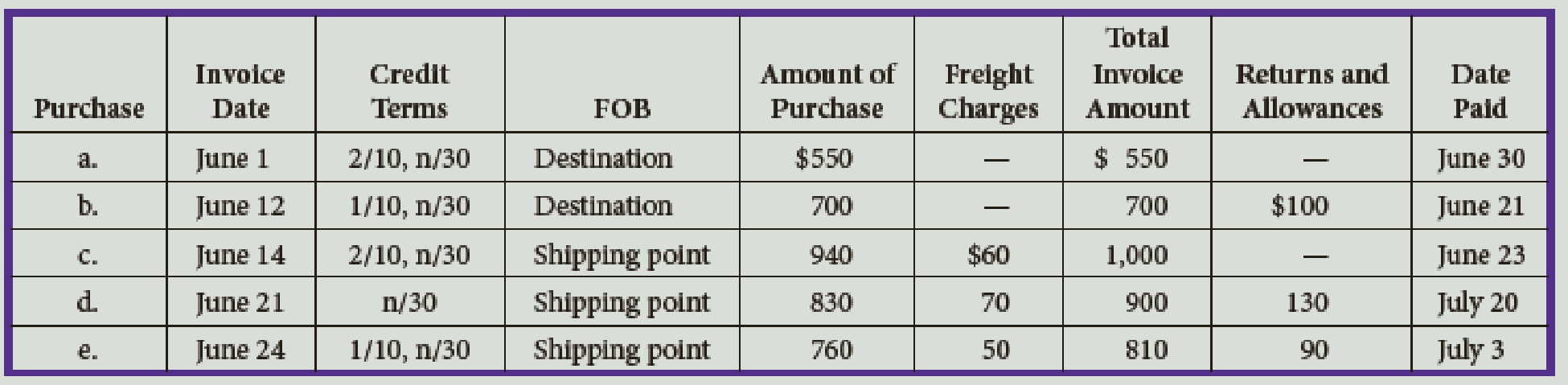# For the following purchases of merchandise, determine the amount of cash to be paid.### College Accounting (Book Only): A ...

13th Edition
Scott + 1 other
Publisher: South-Western College Pub
ISBN: 9781337280570### College Accounting (Book Only): A ...

13th Edition
Scott + 1 other
Publisher: South-Western College Pub
ISBN: 9781337280570

#### Solutions

Chapter
Section
Chapter 10, Problem 1E
Textbook Problem

## For the following purchases of merchandise, determine the amount of cash to be paid.Expert Solution
To determine

Calculate the amount of cash to be paid for purchase of merchandise.

### Explanation of Solution

a. Calculate the payment of cash for purchase of merchandise.

The purchase of merchandise is $550 because cash is not paid within the discount period. b. Calculate the payment of cash for purchase of merchandise. Calculate the net purchase. Net Purchase=(Amount of purchasePurchase returns and allowances)=$700$100=$600

Calculate the purchase discount.

Purchase Discount=(Net purchase ×Trade discount)=$600×1%=$6

Calculate the purchase payment.

Purchase Payment =(Net purchasePurchase discount)=$600$6=$594 c. Calculate the payment of cash for purchase of merchandise. Calculate the total invoice amount. Total Invoice Amount=(Amount purchase+Freight charges)=$940+$60=$1,000

Calculate the Purchase Discount

Purchase Discount =(Amount purchase ×Trade discount)=$940×2%=$18.80

Calculate the Purchase payment.

Purchase Payment=(Amount of purchase Purchase Discount)=\$1,00018

### Want to see the full answer?

Check out a sample textbook solution.See solution

### Want to see this answer and more?

Bartleby provides explanations to thousands of textbook problems written by our experts, many with advanced degrees!

See solution

Find more solutions based on key concepts
Show solutions
Define IP spoofing.

Accounting Information Systems